# Length of the arc

What is the length of the arc of a circle k (S, r=68mm), which belongs to a central angle of 78°?

x =  92.5723 mm

### Step-by-step explanation: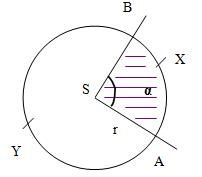Did you find an error or inaccuracy? Feel free to write us. Thank you!Tips to related online calculators
Do you want to convert length units?

#### You need to know the following knowledge to solve this word math problem:

We encourage you to watch this tutorial video on this math problem:

## Related math problems and questions:

• Central angleWhat is the length of the arc of a circle with a diameter of 46 cm, which belongs to a central angle of 30°?
• The central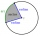The central angle of a sector is 30° and the radius is 15 m. Determine its perimeter.
• ArcCircle arc corresponding to angle is 32° is 28 dm long. What is the length of the entire circle?
• Circle and angleWhat is the length of the arc of a circle with radius r = 207 mm with cental angle 5.33 rad?
• Arc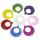What area of a circle occupied the flowers planted in the arc of a circle with radius 3 m with central angle 45°?
• Circular arcCalculate the length of the circular arc if the diameter d = 20cm and the angle alpha = 142 °
• Quarter circularThe wire that is hooked around the perimeter of quarter-circular arc has length 3π+12. Determine the radius of circle arc.
• The length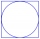The length of the circle is 24 cm. What is the length of the circular arc of the corresponding angle 30°?
• The two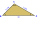The two angles of a triangle are 78° and 82°. So what is the measure of the remaining third angle?
• Track arc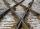Two straight tracks is in an angle 74°. They will join with circular arc with radius r=1127 m. How long will be arc connecting these lines (L)? How far is the center point of arc from track crossings (x)?
• Arc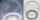The length of the circle is 41 amd arc length of the circle 9. What is the magnitude of the angle of this arc?
• Circle arcCalculate the circular arc area in m2 where the diameter is 263 dm, and a central angle is 40°. Result round to three decimal places.
• TrianglesFind out whether given sizes of the angles can be interior angles of a triangle: a) 23°10',84°30',72°20' b) 90°,41°33',48°37' c) 14°51',90°,75°49' d) 58°58',59°59',60°3'Calculate the radius of the quadrant, which area is equal to area of circle with radius r = 15 cm.Calculate the length of circular arc l, area of the circular arc S1 and area of circular segment S2. Radius of the circle is 11 and corresponding angle is (2)/(12) π.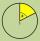The circumference of the disk is 78.5 cm. What is the circumference of the circular arc of 32° on the disc?Circle segment has a circumference of 135.26 dm and 2096.58 dm2 area. Calculate the radius of the circle and size of the central angle.